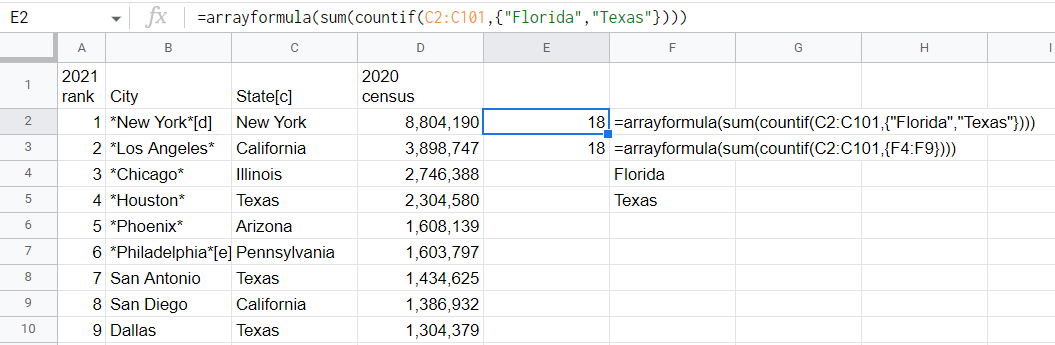# Count Cells with Specific Text in Google Sheets (The Easy Way!)

5 MinutesAfter learning how COUNTIF works in Google Sheets, you will find that there are numerous possibilities.

## Count Cells with a Specific Text using the COUNTIF Function

### Syntax

=COUNTIF(range,”text”)

Where

text is the text you are looking for

range is where you want to look for the text

### Step 1: Identify the text and range

We want to count cities of Florida in our list of cities with their corresponding states. The range is C2:C101 and the text is “Florida”.

### Step 2: Select the cell, then add the formula with the format =COUNTIF(range,”text”)

The formula will now be

=countif(C2:C101,"Florida")

That’s it! You can now tally cells with a certain text using COUNTIF. Let us apply it to more cases.

## Count Number of Cells that DO NOT Contain a Specific Text

### Syntax

=COUNTIF(range,”<>text”)

Where

text is the text you want to exclude

range is where you want to look for the text

The <> symbol means not equal.

### Step 1: Identify the text and range

We want to tally cities that are not in California. The range is C2:C101 and the text is “<>California”.

### Step 2: Select the cell, then add the formula with the format =COUNTIF(range,”text”)

The formula will now be

=countif(C2:C101,"<>California")

## Count number of Cells that Contain a Portion of a String

Wildcard symbols tell Google Sheets that a set of characters precede or follow a given string. We use asterisk * as it means the preceding or following characters have varying lengths.

### Syntax

##### When you know the first part of the text

=COUNTIF(range,”string*”)

##### When you know the last part of the text

=COUNTIF(range,”*string”)

##### When you know the middle part of the text

=COUNTIF(range,”*string*”)

This will also count cases where the string appears at the start or at the end.

### Step 1: Identify the text and range

We want to count cities whose states they belong to have the string “ia” in their name: at the beginning, in the middle, and at the end of their names. The range is C2:C101 and the string for each case are:

“ia*” for cells with “ia” at the start

“*ia*” for cells with “ia” at the middle

“*ia” for cells with “ia” at the end

### Step 2: Select the cell, then add the formula with the format =COUNTIF(range,”text”)

See? It’s straightforward!

## Count Cells with 2 or More Given Texts using the COUNTIF Function

You can determine how frequently two or more given texts appear in the selected range by making a combination of COUNTIF with ARRAYFORMULA and SUM functions.

### Syntax

=ARRAYFORMULA(SUM(COUNTIF(range,{”text1”,”text2”})))

Where

text1 and text2 are the strings you are tallying

range is where you want to look for text1 and text2

### Step 1: Identify the text and range

We want to count cities that are located in Texas and Florida. The range is C2:C101, and text1 and text2 are “Texas” and “Florida”, respectively.

### Step 2: Select the cell, then add the formula with the format =ARRAYFORMULA(SUM(COUNTIF(range,{”text1”,”text2”})))

For our example, the formula will be

=arrayformula(sum(countif(C2:C101,{"Florida","Texas"})))Count if cell contains given text google sheets, find instances of cells that has two or more texts

## FAQs

### Can the string be stored in another cell?

Yes! It’s generally straightforward to do so. The syntax is

=COUNTIF(range, cell_containing_text)

Where

cell_containing_text is the reference to the cell containing the text

range is where you want to look for the text

The difference here is that the text stored in another cell no longer needs to be enclosed in double quotes.

For counting the number of cells that do not contain a certain text, you need to use CONCATENATE function:

=COUNTIF(range,CONCATENATE(“<>”,cell_containing_text)